Warning

# Convolutional Neural Networks

In this lab, we: (i) learn how to make a simple neural network (NN) in a pure NumPy, and (ii) build and train Convolutional Neural Networks (CNN) using the PyTorch framework. In particular, we will train several different CNN classifiers of handwritten digits (0-9) from 28×28 MNIST grayscale images.

Today, there are many resources available about deep learning and CNNs. You can find some useful ones at the bottom of this page. However, hold on with reading them all at first. In this lab, we are going slowly. We add one component after another. There are links to relevant explanations in the text itself, so whenever you meet a new term, you also learn its meaning. You may want to revisit these links or check some other resources after finishing the lab.

## Information for development

To fulfil this assignment, you need to submit these files (all packed in a single .zip file) into the upload system:

• numpy_nn.py - containing the following implemented classes:
• ReLU - REctified Linear Unit layer
• Linear - Linear (aka Fully Connected) layer
• Sigmoid - Logistic Sigmoid layer
• SE - Squared Error loss layer
• numpy_nn_training.png, numpy_nn_classification.png
• pytorch_cnn.py - containing the following implemented classes and methods:
• MyNet - Your CNN model
• classify - classifies data using a trained network
• model.pt - trained MyNet state

Use template of the assignment. When preparing a zip file for the upload system, do not include any directories, the files have to be in the zip file root.

The challenge: The network will be automatically evaluated by the upload system and ranked in the online score board. The gained points from the assignment depend on the rank of your algorithm (assuming your code passes all the AE tests):

• 1st place: 16 points
• 2nd place: 14 points
• 3rd place: 12 points
• 4th place: 10 points
• every submission with performance worse than the baseline: 0 points
• 8 points otherwise

Deadline for the submission is Sun Jan 12 23:59. Then the points will be assigned. Every later submission is then for 6 points. You have to be better than the baseline to complete this lab.

## 1. Simple Neural Network in NumPy

We start by implementing few simple layers in pure NumPy to get the understanding of what is going on in the machine learning frameworks like PyTorch, TensorFlow, etc.

Recall, that a neural network is an acyclic graph with individual nodes being either simple Perceptrons (with non-linearity) or other 'layers'. A layer is an object which has two main functionalities: (i) it can pass the data forward during the prediction/classification phase – implemented in layer.forward method, and (ii) it computes gradients with respect to (w.r.t.) its inputs which are then passed backward during back-propagation training – implemented in layer.backward method. This allows to chain many layers into possibly large graphs and then compute efficiently the gradient of the loss function w.r.t. every parameter.

We recommend very much the lecture by Karpathy for a clear explanation of the gradient propagation during back-propagation training. In short, one starts first by propagating the input data through the forward calls in each layer sequentially from the first to the last layer. Then, starting at the last layer, one computes the gradients (with the backward function) and passes them to the preceding layers. This is then repeated in every layer. After all the gradients are computed, we train the network by gradient descent.

You will see that out of all layers which we consider, only the fully-connected layer (or linear layer as we call it) has some trainable parameters. During training, we update by the partial derivatives of the loss w.r.t. the parameters of the network. You might consider the parameters as another extra input of the layer (along with the outputs from the previous layer). For the details, see the Karpathy's lecture.

For a layer with trainable parameters, there is also a method layer.grads which returns the gradients of the loss w.r.t. its parameters.

Implement the following layers.

### Fully-Connected (Linear) layer

In the forward method, the Linear layer implements the function $f(\mathbf{x}) = \mathbf{W} \mathbf{x} + \mathbf{b}$. Hint: You may need to store the inputs $x$ for later use in the backward method.

In the backward method, the gradient of the layer w.r.t. $\mathbf{W}$ and $\mathbf{b}$ is computed. The method returns the gradient of the loss w.r.t. the layer's input. Both forward and backward methods must work with several data samples stacked together (these are called batches or minibatches). Make sure that you check your shapes against the docstring specifications! Remember, CHECK YOUR SHAPES! Reshaping, adding extra dimensions or transposing the arrays may come handy (you might want to check the NumPy - HOW TO thread at the forum).

Make sure that the gradients' computation is working for batched data and their shapes are the same as the docstring tells you. If you are getting a weird error in BRUTE, it is very likely that your shapes are wrong!

### ReLU non-linearity

ReLU is a commonly used non-linear layer which computes $f(\mathbf{x}) = \text{max}(\mathbf{0}, \mathbf{x})$. Both forward and backward methods are much simpler as the layer has no parameters.

Make sure it works for arbitrarily shaped inputs (even 3D, 4D, or more-D)!

And again, you will need to remember what happened during the forward pass in order to compute the gradient correctly.

### Sigmoid non-linearity

You already know the logistic sigmoid from the logistic regression lab. The Sigmoid layer implements the function $f(\mathbf{x}) = \frac{1}{1 + e^{-\mathbf{x}}}$. As in the ReLU layer, there are no parameters in the Sigmoid layer. The backward method only computes the gradient of the loss w.r.t. the layer inputs.

### Squared Error Loss

The squared error loss computes $(\mathbf{x}-\mathbf{y})^2$. The backward pass computes the gradient of the loss only w.r.t. the input $x$.

## Defining and training the network

Your task is to train a network for a binary classification of two selected classes (numbers) from the MNIST dataset. The data loading, model definition, and training is done in the if __name__ == '__main__':  section of the numpy_nn.py template.

Experiment with the hyper-parameter settings (Slow training? Try increasing learning_rate. No convergence? Try decreasing learning_rate) and the model architecture. A typical model has several layers organised as Linear → ReLU → Linear → ReLU → … → Linear → Sigmoid, but many other options are possible.

1. Does adding more fully-connected layers help?
2. Does having more output units in the fully-connected layer help?
3. What activation functions work the best?
4. Experiment with the batch size and watch how it influences the training.
5. Feel free to implement also another layers and non-linearities.

Save the training images as numpy_nn_training.png and numpy_nn_classification.png

## 2. PyTorch Network

Our simple NumPy NN works well for a simple two digit classification problem, but if we want to solve a more difficult task, it quickly becomes inefficient and then it is better to use one of the highly optimized public libraries. We will work with the currently most popular CNN framework - PyTorch. Writing the neural network using PyTorch is straightforward - there are many layers readily available and you can operate on the data passing through the network just like you would in plain NumPy.

A template for this part of the assignment is in pytorch_cnn.py.

## PyTorch Instalation

Start by installing PyTorch - follow the instructions at https://pytorch.org/get-started/locally/.

## Problem specification

Your task is to classify images of hand-written digits 0-9. Fortunately, there is quite a lot of annotated data readily available on the internet: MNIST. To simplify loading the data, use torchvision MNIST dataset which downloads and prepares the data for you (see the template).

Unfortunately, during the testing phase, your customer (a company called BRUTE & SON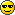) is using a low quality camera with lot of noise of unknown characteristics. So, even though the hand written style of the MNIST dataset is representative enough, the images are heavily corrupted (see examples below). We do not need to mention that the camera is proprietary and you will never have a chance to test it on your images, do we?

Your only chance is to use the data augmentation technique and try to mimic the effect of the camera. You may also try training without augmentation, of course, but we have tried and failed already. That's why we are hiring you after all ;)

Examples of the clean MNIST data: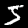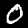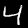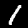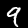Examples of the noisy data from the camera: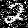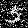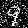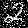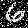## 3. Linear classifier, log-softmax regression, stochastic gradient descent

Lets start with a simple one layer fully-connected network. You should now understand how it works under the hood after the first part of this assignment. Here we will need to extend the binary classification to the multi-class one. Fortunately, this is quite easy using a softmax layer.

The template contains a code for training a simple one layer network FCNet with a log-softmax regression on the output and trained using the stochastic gradient descent.

1. Train the FCNet network on the MNIST data (see the train function in the template)

2. Add another fully-connected layer with 1000 hidden units and with sigmoid non-linearity. Do you get better results?

3. Try to add more layers. Does the network improve with more and more layers?

4. How many weights do you learn in each case?

5. Try to substitute the sigmoid non-linearity with Rectified Linear Unit (ReLU). It helps to avoid the vanishing gradient problem.

6. Experiment with the number of layers, number of hidden units and try to get the best possible result.

## 4. Convolutional Neural Networks

One of the main disadvantages of using the fully-connected layers on images is that they do not take into account the spatial structure of the image. Imagine that you randomly perturb spatial arrangement of image pixels (in both training and test data) and re-train the fully-connected network. These perturbed images become completely unlearnable for humans, since humans make a prior assumption about the spatial arrangement. Nevertheless, the expected testing error of the re-trained network on this randomly perturbed dataset will be the same, since it does not make any assumptions and learns the spatial arrangement from the scratch from perturbed training data. When we learn on images, architecture of the network should reflect the particular spatial arrangement.

We impose the spatial arrangement by introducing the convolutional layers. The convolution works as shifting a local template (often called a convolution kernel or a local receptive field) over the image and computing its response for every single position in the image. For example, when the input image is 28×28, and we compute convolution with 5×5 kernel, than resulting response image will be 24×24 (unless we pad the image with zeros). When learned, these templates often corresponds to edge or corner detectors.

Another disadvantage of the fully-connected layers is that the number of parameters grows quickly with new layers. This means significantly more parameters need to be learned and thus more data need to be used to avoid overfitting.

1. Train a CNN with one convolutional layer (3×3 kernel) followed by a ReLU non-linearity and a fully connected layer with log-softmax criterion (see SimpleCNN provided in the template).

Notice that the kernel is not evaluated at every position but at every second only (stride=2). This makes the second layer smaller while keeping most of the information present (as nearby convolutions result in similar values). We also added padding=1, which adds zeros to the image before computing the convolution. This way, the size of the output stays the same when stride=1 and becomes half when stride=2.

2. Are you getting better results than with fully connected networks? Does taking into account the spatial arrangement help?

3. How many parameters are you learning now? Compare this to the case of the two layered fully connected network.

4. Add one more convolutional layer with ReLU (again with stride=2).

5. Visualise the learned filters in the first convolutional layer (they are 3×3 matrices).

6. To train a CNN one still needs a lot of data as the number of parameters being estimated is large. To avoid over-fitting, two another techniques are commonly used: max-pooling and dropout. Substitute the stride=2 by stride=1 and max-pooling and add a dropout layer before the fully connected layer.

7. Experiment :) Your goal is to train the best possible network (implement it in MyNet and submit it to the upload system for the competition. You can play with:
• longer training
• number of layers
• number and size of the convolutional kernels
• batch size
• data augmentation (to get more data)
• pre-training the network on another (larger) dataset
• learning rate schedule